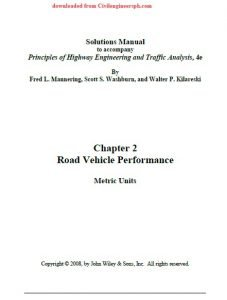# Solutions Manual Principles of Highway Engineering & Traffic AnalysisSolution Manual Principles of Highway Engineering and Traffic Analysis, 4e
By
Fred L. Mannering, Scott S. Washburn, and Walter P. Kilareski

in Metric units as well as U.S. Customary Units both.

Preface
The solutions to the fourth edition of Principles of Highway Engineering and Traffic Analysis were prepared with the Mathcad1 software program. You will notice several notation conventions that you may not be familiar with if you are not a Mathcad user. Most of these notation conventions are self-explanatory or easily understood. The most common Mathcad specific notations in these solutions relate to the equals sign. You will notice the equals sign being used in three different contexts, and Mathcad uses three different notations to distinguish between each of these contexts. The differences between these equals sign notations are explained as follows.

The ‘:=’ (colon-equals) is an assignment operator, that is, the value of the variable or expression on the left side of ‘:=’is set equal to the value of the expression on the right side. For example, in the statement, L := 1234, the variable ‘L’ is assigned (i.e., set equal to) the value of 1234. Another example is x := y + z. In this case, x is assigned the value
of y + z.
• The ‘=’ (bold equals) is used when the Mathcad function solver was used to find the value of a variable in the equation. For example, in the equation , the = is used to tell Mathcad that the value of the expression on the left side needs to equal the value of the expression on the right side. Thus, the Mathcad solver can be employed to find a value for the variable ‘t’ that satisfies this relationship. This particular example is from a problem where the function for arrivals at some time ‘t’ is set equal to the function for departures at some time ‘t’ to find the time to queue clearance.
• The ‘=’ (standard equals) is used for a simple numeric evaluation. For example, referring to the x := y + z assignment used previously, if the value of y was 10 [either by assignment (with :=), or the result of an equation solution (through the use of =) and the value of z was 15, then the expression ‘x =’ would yield 25. Another example would be as follows: s := 1800/3600, with s = 0.5. That is, ‘s’ was assigned the value of 1800 divided by 3600 (using :=), which equals 0.5 (as given by using =).

Book Principles of Highway Engineering and Traffic Analysis By Fred L. Mannering and Scott S. Washburn also available here.

Solutions Manual Principles of Highway Engineering & Traffic Analysis, Solutions Manual Principles of Highway Engineering & Traffic Analysis, Solutions Manual Principles of Highway Engineering & Traffic Analysis, Solutions Manual Principles of Highway Engineering & Traffic Analysis, Solutions Manual Principles of Highway Engineering & Traffic Analysis, Solutions Manual Principles of Highway Engineering & Traffic Analysis, Solutions Manual Principles of Highway Engineering & Traffic Analysis, Solutions Manual Principles of Highway Engineering & Traffic Analysis, Solutions Manual Principles of Highway Engineering & Traffic Analysis, Solutions Manual Principles of Highway Engineering & Traffic Analysis

## 12 Replies to “Solutions Manual Principles of Highway Engineering & Traffic Analysis”

1.eric on said:

thank you

•CIVILENGINEERSPK on said:

For Members only

2.abdulhalim bilgin on said:

can ı dowloand principles of highway engineering and traffic analysis 5th edition solution manual civil. please

•CIVILENGINEERSPK on said:

3.sethu on said:

•CIVILENGINEERSPK on said:

Thats not possible. You should be signed in by ur gmail id to download anything. If you are unable to send us the link which you are unable to download at civilengineerspk@gmail.com and we will send it to you.

4.Brian Bravenboer on said:

Good day,
Is it possible that I can download “Principles of highway engineering and traffic analysis 5th edition solution manual please (Metric Units).
Kind Regards,
Brian

•CIVILENGINEERSPK on said:

5.Brian Bravenboer on said:
6.Zahraa on said:
•CIVILENGINEERSPK on said: